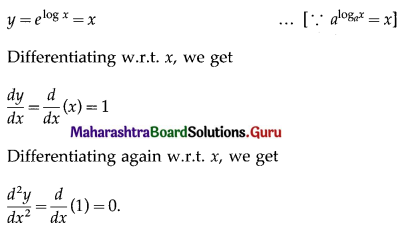Balbharati Maharashtra State Board 12th Commerce Maths Solution Book Pdf Chapter 3 Differentiation Ex 3.6 Questions and Answers.

## Maharashtra State Board 12th Commerce Maths Solutions Chapter 3 Differentiation Ex 3.6

1. Find $$\frac{d^{2} y}{d x^{2}}$$ if,

Question 1.
y = √x
Solution: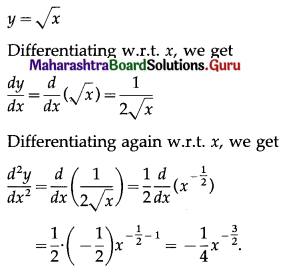Question 2.
y = x5
Solution: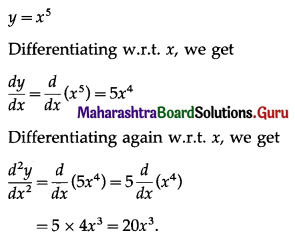Question 3.
y = x-7
Solution: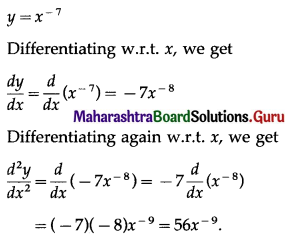2. Find $$\frac{d^{2} y}{d x^{2}}$$ if,

Question 1.
y = ex
Solution: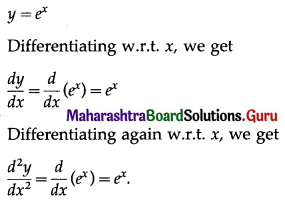Question 2.
y = e(2x+1)
Solution: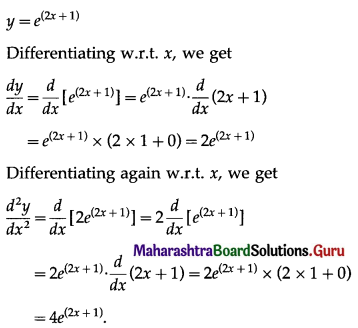Question 3.
y = elog x
Solution: